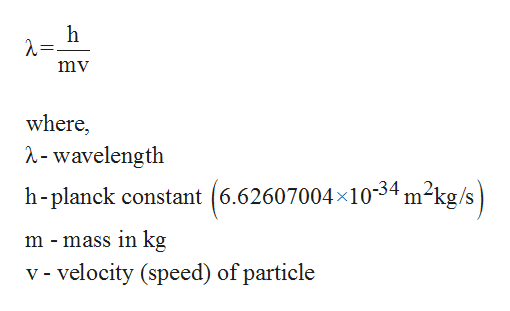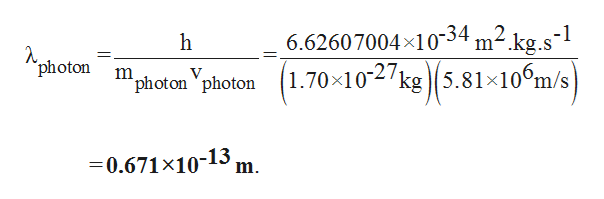Answer should be in meters. Calculate the wavelength of:a proton (1.70×10-27 kg) moving at a speed of 5.81×106 m/s (13000000 miles per hour).a bullet (1.90×10-3 kg) moving at a speed of 313 m/s (700 miles per hour).

Question

Answer should be in meters. Calculate the wavelength of:

 a proton (1.70×10-27 kg) moving at a speed of 5.81×106 m/s (13000000 miles per hour). a bullet (1.90×10-3 kg) moving at a speed of 313 m/s (700 miles per hour).
Step 1

Using De-Broglie wavelength formula, the wavelength is calculated.help_outlineImage Transcriptioncloseh mv where, ^-wavelength h-planck constant (6.62607004x1034 m2kg/s m - mass in kg v - velocity (speed) of particle fullscreen
Step 2

The wavelength of proton from the ...help_outlineImage Transcriptionclose6.62607004x1034 m2.kg.s-1 (1.70 102kg5.81x106m/s h photon 'photon 'photon =0.671x10-13 m. fullscreen

Want to see the full answer?

See Solution

Want to see this answer and more?

Our solutions are written by experts, many with advanced degrees, and available 24/7

See Solution
Tagged in

General Chemistry